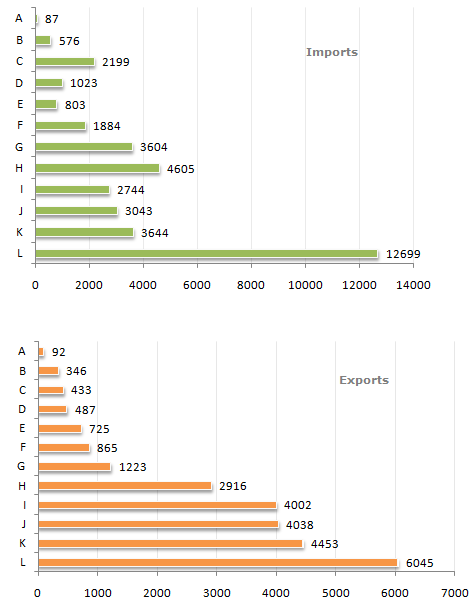# Data Interpretation - Bar Charts - Discussion

Study the following bar charts and answer the questions.

Foreign Trade (Imports and Exports) by countries for the year (1993 - 1994)3.

The total trade deficit/surplus for all the countries put together was ?

 [A]. 11286 surplus [B]. 11286 deficit [C]. 10286 deficit [D]. None of these

Explanation:

Sum of exports - Sum of imports = deficit(11286).

 Divya said: (Jan 27, 2014) Please explain in detail how to calculate deficit and surplus? I can't understand this whole bar chart. Please help me out in all 5 pbms.

 Piyush said: (Aug 12, 2014) For each company say "A". Since export is more than import in this case, therefore it is surplus for the company i.e = 92-87 = 5. So, if export > import, it is surplus else it is deficit. :).

 Abc said: (Aug 13, 2014) Please explain the concept of surplus and deficit. Also the scale for both the graphs is different.

 Amandeep said: (Aug 2, 2015) How can I simply this question? Adding so many values can waste a lot of time.

 Spatel said: (Aug 21, 2015) Surplus = IE. Deficit means less than enough and surplus means more than necessary so we can say surplus only happen when I

 Shraddha said: (Jun 23, 2017) If total import > total export then deficit. Total export > total import then surplus.

 Ashwini said: (Aug 7, 2018) When comparing both the graph if import > export then its deficit. Deficit= sum of export - sum of import. =25625-36911 = 11286 deficit. Hence answer is B.

 Meenu said: (Dec 20, 2019) Is there any short trick?

 Deepu said: (Mar 15, 2020) There is no short trick.